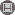Volume 6, Issue 4 (16 2011)                   irje 2011, 6(4): 28-39 | Back to browse issues page

BibTeX | RIS | EndNote | Medlars | ProCite | Reference Manager | RefWorks
Send citation to:Sedehi M, Mehrabi Y, Kazemnejad A, Joharimajd V, Hadaegh F. Artificial Neural Network Design for Modeling of Mixed Bivariate Outcomes in Medical Research Data. irje. 2011; 6 (4) :28-39
URL: http://irje.tums.ac.ir/article-1-66-en.html
Abstract:   (11642 Views)
Background & Objective: Mixed outcomes arise when, in a multivariate model, response variables measured on different scales such as binary and continuous. Artificial neural networks (ANN) can be used for modeling in situations where classic models have restricted application when some of their assumptions are not met. In this paper, we propose a method based on ANNs for modeling mixed binary and continuous outcomes.
Methods: Univariate and bivariate models were evaluated based on two different sets of simulated data. The scaled conjugate gradient (SCG) algorithm was used for optimization. To end the algorithm and finding optimum number of iteration and learning coefficient, mean squared error (MSE) was computed. Predictive accuracy rate criterion was employed for selection of appropriate model. We also used our model in medical data for joint prediction of metabolic syndrome (binary) and HOMA-IR (continues) in Tehran Lipid and Glucose Study (TLGS). The codes were written in R 2.9.0 and MATLAB 7.6.
Results: The predictive accuracy for univariate and bivariate models based on simulated dataset Ι, where two outcomes associated with a common covariate, were shown to be approximately similar. However, in simulated dataset ΙΙ in which two outcomes associated with different covariates, predictive accuracy in bivariate models were seen to be larger than that of univariate models.
Conclusions: It is indicated that the predictive accuracy gain is higher in bivariate model, when the outcomes share a different set of covariates with higher level of correlation between the outcomes.